# Odds and Ends in Haskell Folding IO and

• Slides: 23
Download presentationOdds and Ends in Haskell: Folding, I/O, and Functors Adapted from material by Miran Lipovaca 0lists: - base case on empty list The function - somefoldl operation with the head, plus a recursive call on the tail This is such a common pattern that there is a higher-order function to handle it. Inputs: a function, a initial starting value (which we’ll call the accumulator, although it can have any name) and a list to “fold up” 1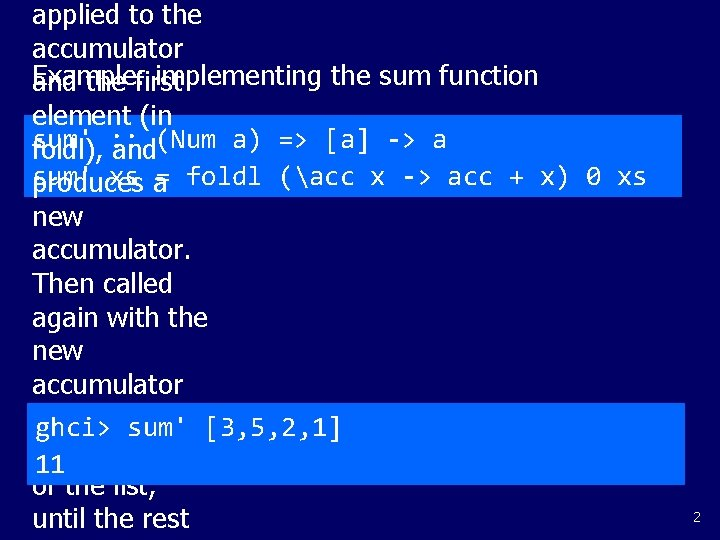applied to the accumulator Example: implementing the sum function and the first element (in sum' : : (Num a) => [a] -> a foldl), and sum' xs = foldl (acc x -> acc + x) 0 xs produces a new accumulator. Then called again with the new accumulator and the new ghci> sum' [3, 5, 2, 1] first 11 element of the list, until the rest 2The lambda In fact, we can write this function in an even shorter function onfunctions can be returned as parameters: way, since the previous slide is really sum' : : (Num a) => [a] -> a the same as sum' = foldl (+) 0 (+), and we can omit xs because the function written above will just return a function that takes a list as input. 3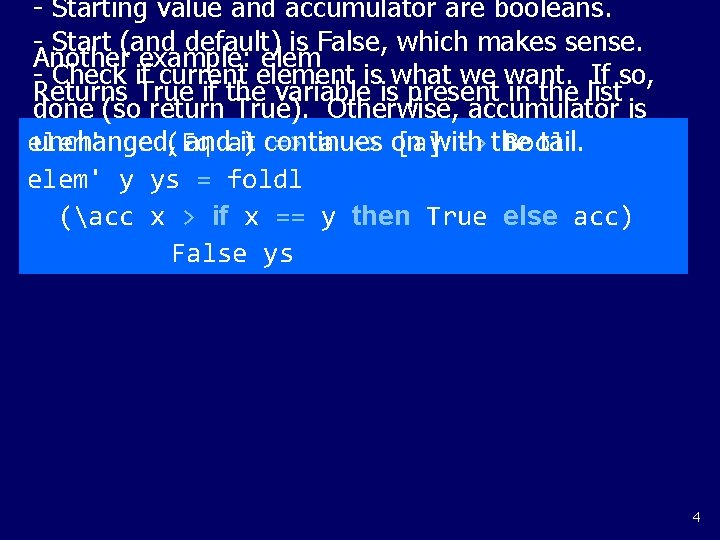- Starting value and accumulator are booleans. - Start (and default) is False, which makes sense. Another example: elem - Check if current element is what we want. If so, Returns True if the variable is present in the list done (so return True). Otherwise, accumulator is elem' : : (Eq a) => a -> [a] -> Bool unchanged, and it continues on with the tail. elem' y ys = foldl (acc x > if x == y then True else acc) False ys 4function). - Scanl and scanr work just the same, but return all intermediate accumulator values in a list. - foldl 1 and foldr 1 work just the same as foldl and foldr, but don’t need to provide a starting value they assume first (or last) element of the list is the starting value. 5done very little true input or output. This is logical in a functional language, since nothing has side File effects!I/O However, this is a problem with I/O, since the whole point is to take input (and hence change some value) and then output something (which requires changing the state of the screen or other I/O device. Luckily, Haskell offers work-arounds that separate the more imperative I/O. 6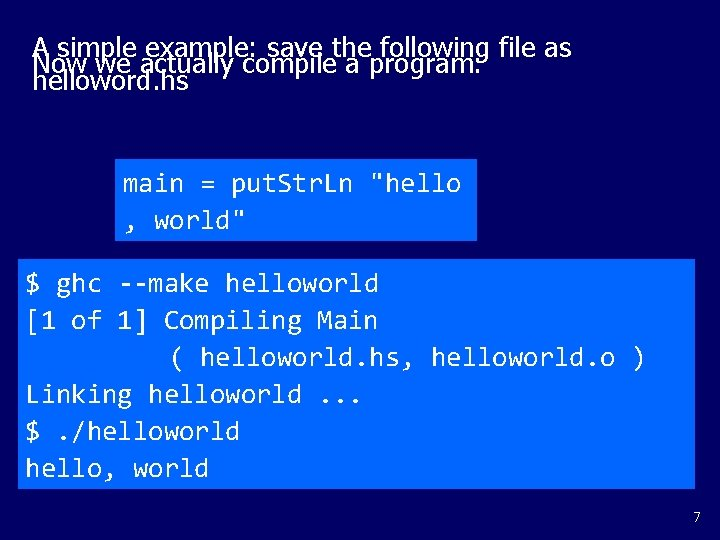A simple example: save the following file as Now we actually compile a program: helloword. hs main = put. Str. Ln "hello , world" \$ ghc --make helloworld [1 of 1] Compiling Main ( helloworld. hs, helloworld. o ) Linking helloworld. . . \$. /helloworld hello, world 7(which has a result type of (), the empty tuple). In Haskell, an I/O action is one with a side effect What are these functions? usually ghci> : t put. Str. Ln either reading or printing. Usually some kind put. Str. Ln : : String of a return value, where () is a dummy value for no return. > IO () ghci> : t put. Str. Ln "hel lo, world" put. Str. Ln "hello, world " : : IO () 8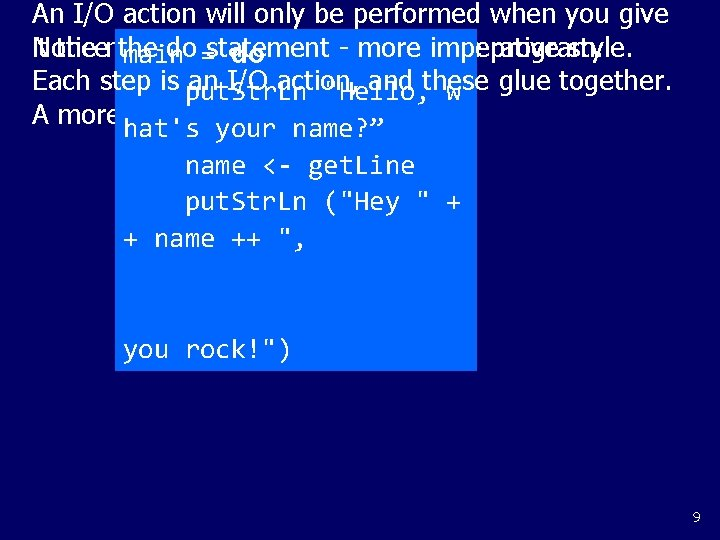An I/O action will only be performed when you give Notice the do statement - more style. it the name “main” and then runimperative the program. main = do Each step is an I/O action, and these glue together. put. Str. Ln "Hello, w A more hat's your name? ” interesting example: name <- get. Line put. Str. Ln ("Hey " + + name ++ ", you rock!") 9Once the string is returned, we use the <- to bind the result to the specified identifire. More on get. Line: Notice this is the first non-functional action we’ve seen, since this function will NOT have the same ghci> : t get. Line value every time it is run! This is called “impure” get. Line : : IO String code. 10parameters to have the same type. What is theexample: return type of get. Line? An invalid Another word of warning: what does the following do? name. Tag = "Hello, my n name = get. Line ame is " ++ get. Line 11- A main function - inside a bigger I/O block that we have composed with a do (and remember that the last action can’t be bound to a name, since that is the one that is the return type). ghci> put. Str. Ln "HEEY" -At the ghci prompt: HEEY 12main = do put. Str. Ln "What's your You canfirst name? " use let statements inside do blocks, to call Note that <- is for I/O, and let for expressions. other functions (and with no “in” part required): first. Name <- get. Line put. Str. Ln "What's your last name? " last. Name <- get. Line let big. First. Name = map to. Upper first. Name big. Last. Name = map to. Upper last. Name put. Str. Ln \$ "hey " ++ b ig. First. Name ++ " " ++ big. Last. Name ++ ", ho w are you? " 13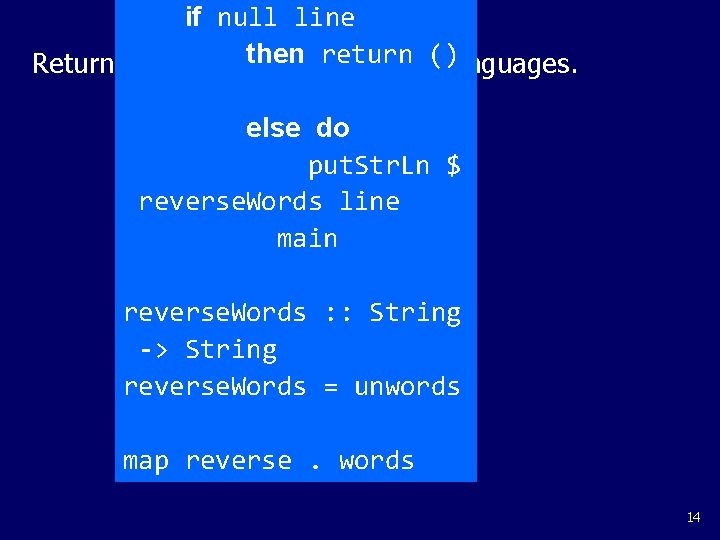if null line Return then return () in haskell: NOT like other languages. else do put. Str. Ln \$ reverse. Words line main reverse. Words : : String -> String reverse. Words = unwords map reverse. words 14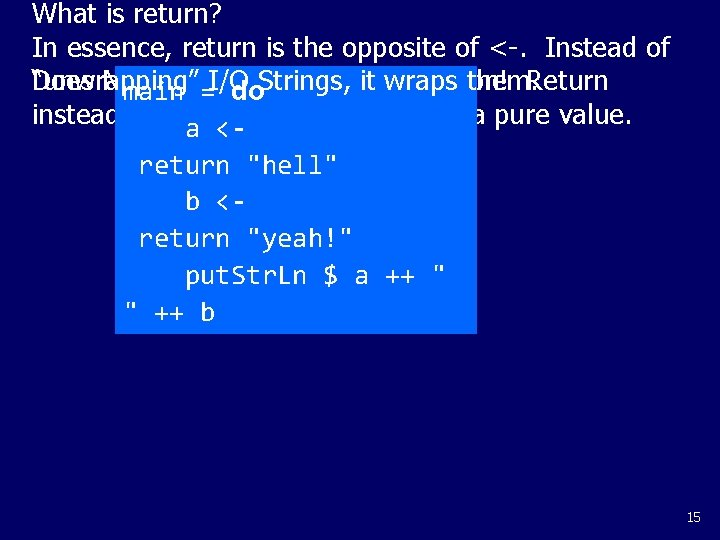What is return? In essence, return is the opposite of <-. Instead of “unwrapping” I/Othe Strings, wraps them. Return Does NOT signal end ofit execution! main = do instead a <makes an I/O action out of a pure value. return "hell" b < return "yeah!" put. Str. Ln \$ a ++ " " ++ b 15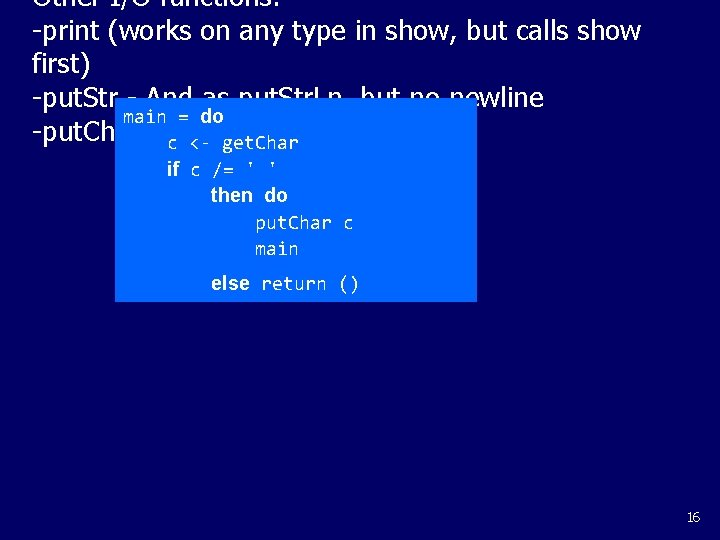Other I/O functions: -print (works on any type in show, but calls show first) -put. Str - And as put. Str. Ln, but no newline main = do -put. Char and get. Char c <- get. Char main = do print True if c /= ' ' print 2 then do print "haha" put. Char c print 3. 2 main print [3, 4, 3] else return () 16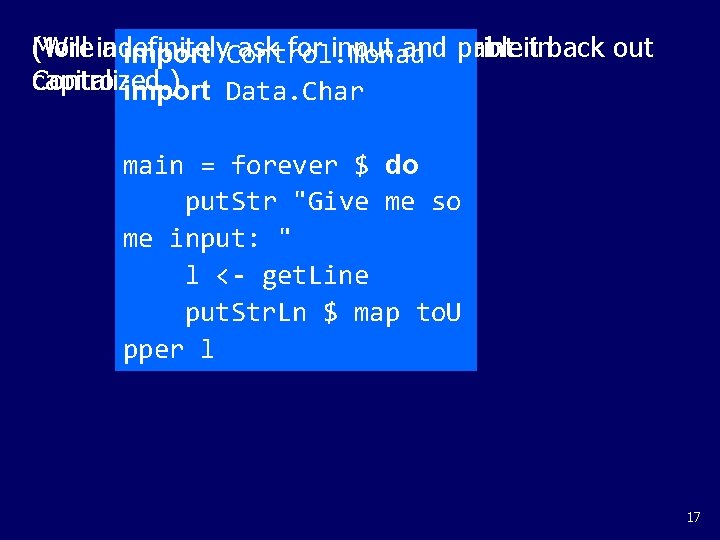(Will indefinitely More advanced functionality ask for inputisand available print itinback out import Control. Monad: capitalized. ) import Data. Char main = forever \$ do put. Str "Give me so me input: " l <- get. Line put. Str. Ln \$ map to. U pper l 17Functors This type are Functors is interesting a typeclass, - not justlikeprevious Ord, Eq, exmaples, Show, and in like all. EQ, the where others. (==) This: : one (Eqisa)designed => a ->toa hold -> Bool. thingsfthat Here, is NOT canabe concrete mappedtype, over; but foraexample, type lists are part of this constructor thattypeclass. takes one parameter. class Functor f where fmap : : (a -> b) -> f a -> f b 18So map is a lot like a functor! Here, map takes a function and a list of type a, and returns a list of Compare fmap to map: type b. In fact, can define map in terms of fmap: fmap : : (a -> b) -> [a] -> [b] > f a -> f b instance Functor [] where fmap = map 19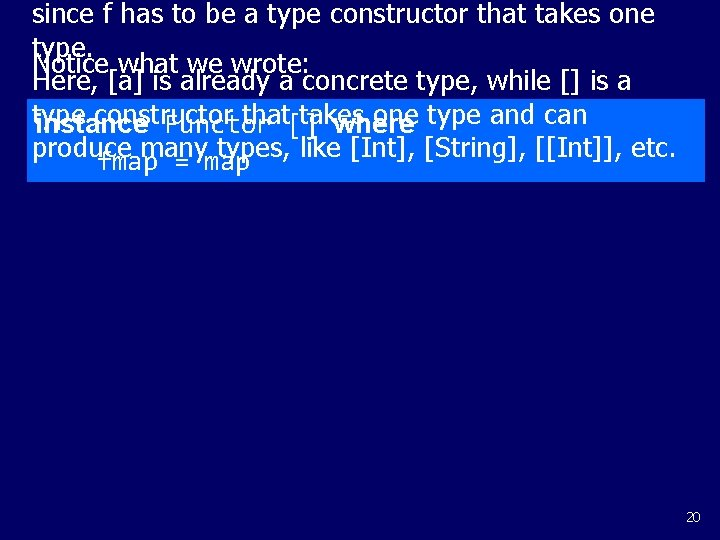since f has to be a type constructor that takes one type. Notice what we wrote: Here, [a] is already a concrete type, while [] is a type constructor that takes one type and can instance Functor [] where produce many types, like [Int], [String], [[Int]], etc. fmap = map 20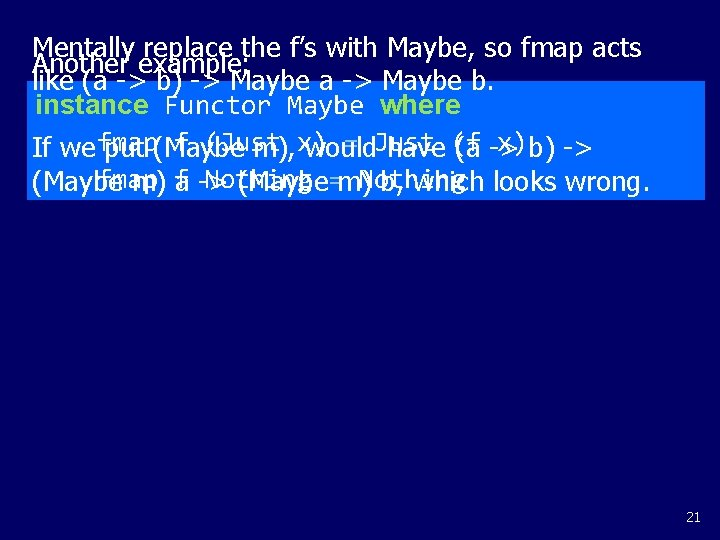Mentally replace the f’s with Maybe, so fmap acts Another example: like (a -> b) -> Maybe a -> Maybe b. instance Functor Maybe where fmap f (Just x) = Just (f x) If we put (Maybe m), would have (a -> b) -> fmap f Nothing = Nothing (Maybe m) a -> (Maybe m) b, which looks wrong. 21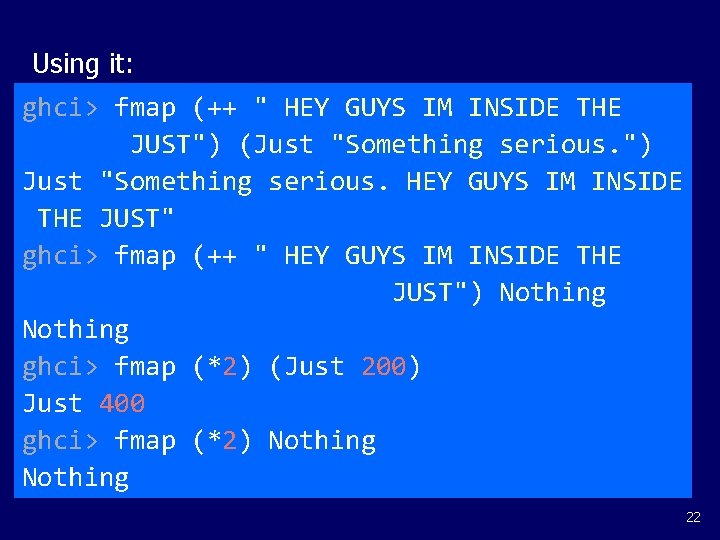Using it: ghci> fmap (++ " HEY GUYS IM INSIDE THE JUST") (Just "Something serious. ") Just "Something serious. HEY GUYS IM INSIDE THE JUST" ghci> fmap (++ " HEY GUYS IM INSIDE THE JUST") Nothing ghci> fmap (*2) (Just 200) Just 400 ghci> fmap (*2) Nothing 22